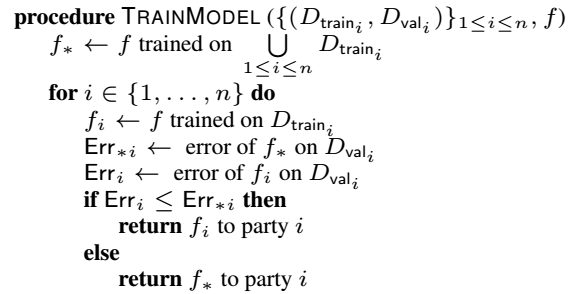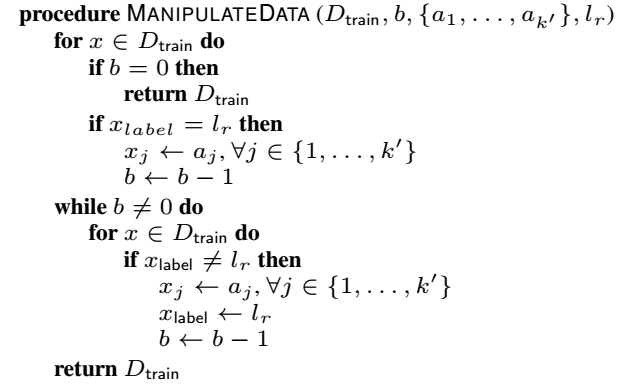# 论文阅读

## Contamination Attacks and Mitigation in Multi-Party Machine Learning

Posted by MY on December 6, 2018

# 概要

本文提出了针对多方合作提供数据集进行训练的深度学习模型进行对抗性攻击，并对这种攻击方法提出了对应的防御方法。

# 简介

多方合作训练是一种有效提高训练效果的方法。例如，多家银行可以分别提供自己银行的客户数据，将数据进行加密处理，放入可以信任的安全训练平台进行训练，从而得到模型来判断一个客户的贷款申请是否该被批准。每个银行无法看到别的银行的数据，它们只能得到训练后的模型。在训练时，训练平台会训练一个针对所有数据集的模型以及针对每个银行自己数据集的模型。如果公有模型的效果比私有模型的效果好，则返回该私有模型所属者以公有模型，否则返回私有模型。
但是，如果有一家银行心存恶意，它将自己的数据集进行了修改，将性别、种族等敏感信息与拒绝贷款批准相联系。在训练后，整体的模型的验证准确率虽然依旧比每个银行私有的训练模型效果更好，但是当客户信息包含这些敏感信息的时候，这些客户可能就会被使用该公有模型的银行拒绝贷款，而增加了去恶意攻击公有模型的银行进行贷款。
针对这个问题，本文提出了以下几个贡献：

• 定义了符号上述情景的“污染攻击”，使得模型学到在属性和标签之间人工建立的联系。
• 使用对抗训练来减缓这种攻击的效果，即使在并不了解属性与对应标签是否受到攻击的情况下，也可以达到目的。
• 使用对抗训练可以保护每个数据的隐私性，即很难让别人推断了解某数据属于哪一方机构。

# 污染攻击

## 设置

假设有$n$家机构合作，每家机构有一个训练数据集$D_{train_{i}}$，它们都想与其他机构合作训练一个模型。除了训练集，每个机构$i$还有一个私有的验证集$D_{val_{i}}$用来评估最终得到的模型。它们不愿意分享自己的数据集，因此使用了一个中央机器学习服务器$S$来组合数据集、训练模型以及验证模型。该服务器的伪代码如下：$TrainModel$将每家机构的训练集和验证集作为输入$(D_{train_{i}},D_{val_{i}}),1 \leq i \leq n$，模型$f$定义了训练以及优化过程，训练得到一个公有模型$f_{*}$和每家机构的私有模型$f_{i}$。对于机构$i$来说，当模型$f_{*}$的验证误差小于自己私有模型$f_{i}$的验证误差时，公有模型才被释放给该机构。

## 攻击模型

本文的攻击方法不需要利用模型参数，也无法更改其他机构数据集的内容。机构中心存恶意的一家被称作为攻击者。它试图在训练时为攻击者数据集中的数据的某些属性值和一个特定的标签人工建立联系。我们将它们分别称作污染属性和污染标签。当这个模型在使用时，若数据中含有污染属性，则很可能会返回一个污染标签。

## 攻击流程

攻击者构建污染数据的方法如下：它将一个良性数据从自己的数据集中取出，并为它增加污染属性，或者选择某属性值并将对应的标签更改为污染标签。它污染的数据数量取决于多少数据被污染时可能会被检查出来。污染数据的伪代码如下：$ManipulateData$的第一个参数是攻击者的训练模型$D_{train}$，其中每个数据$x$包含$k$个属性。设$x$的第$j$个属性为$x_{j}$，它的标签为$x_{label}$。第$j$个属性的值为$a_{j}$，$x_{label}$的值属于${l_{1},l_{2},…,l_{s}}$。$ManipulateData$的第二个参数是一个正整数$b \leq |D_{train}|$，是污染数据的数量。第三个参数是污染属性值的集合${a_{1},…,a_{k’}}$。第四个参数是污染标签值$l_{r},1 \leq r \leq s$。假设攻击者污染了前$k’ \leq k$个属性。流程中，更新攻击者训练数据的值，以使污染属性和标签之间建立人工连接。 　　衡量攻击成功的标准是返回给受害方的模型是公有模型。给定一个数据集$X$，我们将包含污染属性且被分类到污染标签下的数据的数量与包含污染属性的数据的数量的比值作为污染程度的衡量标准：&\frac{|{x\in X:f_{*}(x)=l_{r}\wedge x_{1}=a_{1}\wedge …\wedge x_{k’}=a_{k’}}|}{|{x\in X:x_{1}=a_{1}\wedge …\wedge x_{k’}=a_{k’}}|}&

# 实验

## 实验结果

由下图可知，攻击达到了预期效果，即验证公有模型和私有模型的准确度时，公有模型准确度要高于私有模型。同时，当污染数据增多时，污染程度越高。##### Share